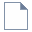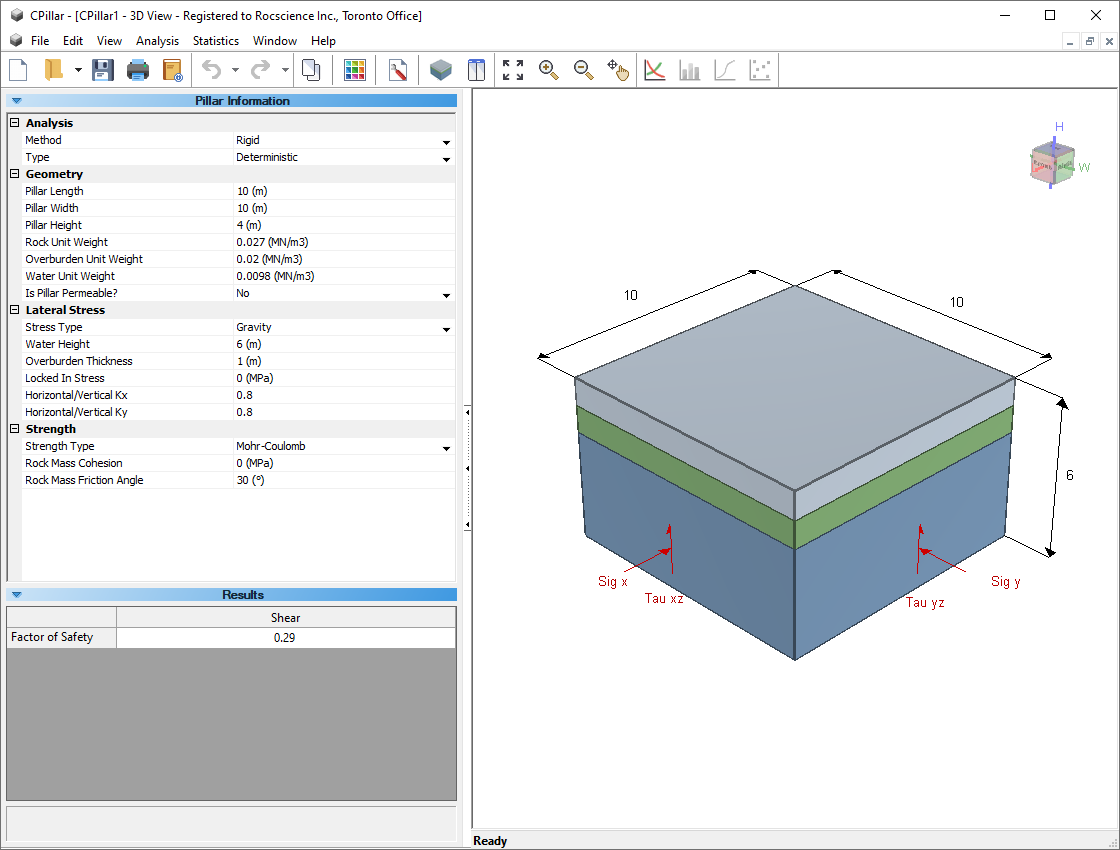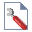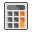The Rocscience International Conference 2021 Proceedings are now available. Read Now

# 3 - Voussoir Analysis of Rectangular Roof Plate

In Tutorial 01 - CPillar Quick Start (Rigid Analysis of Square Pillar), you learned about the Rigid analysis method in CPillar. In Tutorial 02 - Elastic Analysis of Rectangular Roof Beam in CPillar, you learned about the Elastic analysis method. In this tutorial, you will learn about the Voussoir analysis method.

Topics Covered in this Tutorial:

• Project Settings
• Random Variables
• Rectangular Roof Plate Model
• Voussoir Analysis
• Mohr-Coulomb Strength Criterion
• Probabilistic Analysis
• Arch Snap-thru Theory

Finished Product:

The finished product of this tutorial can be found in the Tutorial 3 Voussoir Analysis of Rectangular Roof Plate.cpil5 file, located in the Examples > Tutorials folder in your CPillar installation folder.

## 1.0 Introduction

This model represents a rectangular roof plate (30 x 20 m) with a thickness of 2.5 m.

The Voussoir analysis method will be used. The Voussoir method is applicable for low confining stresses. A span to depth ratio smaller than 3 is not recommended. External lateral stress is not an option for a Voussoir analysis. Lateral stress exists, but it is induced by the ‘arching’ action of the rock, it is not an input parameter.

The Mohr-Coulomb strength criterion will be used. Cohesion is automatically set to zero for a Voussoir analysis when using the Mohr-Coulomb strength criterion. Only a friction angle is entered.

## 2.0 Creating a New File

If you have not already done so, run the CPillar program by double-clicking the CPillar icon in your installation folder or by selecting Programs > Rocscience > CPillar > CPillar in the Windows Start menu.

When the program starts, a default model is automatically created. If you do NOT see a model on your screen:Select: File > New

Whenever a new file is created, the default input data forms valid pillar geometry, as shown in the image below.If the CPillar application window is not already maximized, maximize it now so that the full screen is available for viewing the model. You will have a 3D pillar displayed on the screen in isometric orientation.

## 3.0 Project Settings

The Project Settings dialog allows you to configure the main analysis parameters for your model, such as Units, Analysis Type, and Sampling Method. Select Project Settings on the toolbar or on the Analysis menu.Select: Analysis > Project Settings### 3.1 ANALYSIS TYPE

By default a Deterministic analysis is selected for a new file.

1. Select the General tab.
2. Change the Analysis Type to Probabilistic.

### 3.2 UNITS

In this tutorial we are using metric (MPa) units, so make sure the Metric, stress as MPa option is selected for Units in the Project Settings General tab (default setting).

### 3.3 SAMPLING AND RANDOM NUMBERS

The Sampling Method determines how the statistical distribution for the random input variables will be sampled. The default settings are Sampling Method = Latin Hypercube and Number of Samples = 5,000. For more help, see Sampling in CPillar Project Settings.

### 3.4 PROJECT SUMMARY

Select the Project Summary tab and enter CPillar Voussoir Analysis of Rectangular Roof Plate Tutorial as the Project Title.NOTE: You can have Project Summary information appear on analysis results printouts by setting up a header or footer through Page Setup on the File menu.

• Click OK to close the Project Settings dialog.

## 4.0 Probabilistic Input Data

Autocompute is turned ON by default when performing a Probabilistic Analysis. Autocompute will automatically compute the model every time a change is made in Pillar Information. Let's disable it for now to save on computing time when entering input data.

1. Select: Analysis > Autocompute
2. Uncheck Autocompute for probability analysis
3. Select OKIn CPillar, the entirety of the input parameters are entered in the Pillar Information section of the Sidebar. The Pillar Information section is organized under four headings: Analysis, Geometry, Lateral Stress, and Strength. To change a parameter, click on the value and enter the new value or select from the dropdown as necessary. The model will reflect any changes, immediately.

### 4.1 ANALYSIS

Set up the Analysis:

1. Analysis Method = Voussoir
2. Analysis Type = Probabilistic

### 4.2 GEOMETRY

Enter the following mean data for the pillar geometry parameters:

1. Mean Pillar Length = 30 m
2. Mean Pillar Width = 20 m
3. Mean Pillar Height = 2.5 m
4. Mean Rock Unit Weight = 0.03 MN/m3
5. Mean Overburden Unit Weight = 0 MN/m3
6. Mean Face Dip = 0 deg

NOTE: Water is not included in a Voussoir analysis.

### 4.3 LATERAL STRESS

Enter the following mean data for the stress parameters:

1. Mean Overburden Thickness = 0 m
2. Mean Support Pressure = 0 MPa

### 4.4 STRENGTH

Enter the following mean data for the strength parameters:

1. Strength Type = Mohr-Coulomb
2. Mean Intact UCS = 45 MPa
3. Mean Rock Mass Friction Angle = 30 deg
4. Mean Rock Mass Modulus = 3500 MPa
5. Mean Poisson Ratio = 0.25

### 4.5 RANDOM VARIABLES

In order to perform a Probabilistic analysis, at least one random variable must be assigned a statistical distribution.

The Statistical Distribution is changed by clicking on the distribution iconto the left of the parameter value.

Let's model the following parameters as random variables:

1. Click the distribution iconto the left of Intact UCS, and set:
2. Distribution = Normal

Std. Dev. = 5

Rel. Min and Rel Max = 3x Std. Dev. (Check 3x std. dev.)

3. Click the distribution iconto the left of Mean Rock Mass Friction, and set:
4. Distribution = Normal

Std. Dev. = 5 deg

Rel. Min and Rel Max = 3x Std. Dev. (Check 3x std. dev.)

5. Click the distribution iconto the left of Mean Rock Mass Modulus, and set:
6. Distribution = Normal

Std. Dev. = 500 MPa

Rel. Min and Rel Max = 3x Std. Dev. (Check 3x std. dev.)

7. Click the distribution iconto the left of Mean Poisson Ratio, and set:

Distribution = Normal

Std. Dev. = 0.05 MPa

Rel. Min and Rel Max = 3x Std. Dev. (Check 3x std. dev.)

The Pillar Information side panel should look as follows:NOTE: When using the Mohr-Coulomb Strength Criterion with a Voussoir analysis, the cohesion is automatically set to zero, since by definition the roof is supporting itself through frictional resistance only.

Your model should look like this:## 5.0 Analysis Results

Since we have Autocompute turned OFF, we have to run Compute from the toolbar or on the Analysis menu in order to get the latest analysis results for the current inputs.Select: Analysis > Compute

The primary result from an CPillar Probabilistic analysis are:

• The pillar Mean Factor(s) of Safety (FoS).
• Standard Deviation of the Factor(s) of Safety
• Probability of Failure (expressed as a percentage)

Depending on the Analysis Method (i.e. Rigid, Elastic, or Voussoir), additional data is also presented. The FoS(s) are displayed in the Results section. The Results section appears in the Sidebar on the left side of the CPillar application window and displays a summary of analysis results.

Your results should looks as shown:Note that since this is a Voussoir Analysis, three failure modes are considered:

1. Shear (vertical slippage at the abutments),
2. Arch Snap Thru, and
3. Compression.

The probability of failure is 0.6% under Shear and 0% under Compression. The probability of failure under Arch Snap Thru is Low.

The driving force for Arch Snap-Thru buckling is the self-weight of the rock (i.e. pillar). For a Voussoir Analysis, the Mean Buckling Parameter and Mean Midspan Displacement are also displayed under Arch Snap Thru. The Mean Buckling Parameter represents the percentage of unstable arch configurations for a given geometry and rock mass modulus. A buckling parameter greater than or equal to 35 indicates that the roof is unstable. The 35 corresponds to a midspan deflection of 10% of the beam thickness. This means that arch stability can also be assessed by monitoring the displacement at midspan, relative to the undeflected state.

Note also the Probability of Failure defined as Low. The failure probabilities correspond to mean buckling parameter ranges as indicated below:

 Failure Probability Mean Buckling Parameter Low 0 to 10 Medium 10 to 25 High 25 to 50 Very High greater than 50

Now we will try to predict the critical plate thickness for the collapse of the arch.

## 6.0 Collapse of the Arch

Now let’s gradually collapse our arch by decreasing the plate thickness.

### 6.1 PILLAR HEIGHT = 1 METER

In the Pillar Information side-panel change the pillar height to 1 m. Your results should look as follows:Note that the Shear factor of safety increases, while the Snap-Thru and Compression factors of safety decrease. Note also the increase in the arch Midspan Displacement, from 10.4 mm to 63.5 mm. The Probability of Arch Snap-Thru Failure has increased from Low to Medium.

### 6.2 PILLAR HEIGHT = 0.8 METER

In the Pillar Information side-panel change the pillar height to 0.8 m. Your results should look as follows:Again, Shear factor of safety increases, while Snap-Thru and Compression stability decreases. Arch midspan displacement is now 102 mm. Recall that when the midspan displacement reaches about 10% of the plate or beam height, arch collapse is imminent.

### 6.3 PILLAR HEIGHT = 0.5 METERS

Finally, reduce the pillar height to 0.5 m. Your results should look as follows:The arch is completely unstable, and probability of failure is 100%.

This concludes the tutorial. You can now proceed to Tutorial 04: Empirical Scaled Span Approach in CPillar.